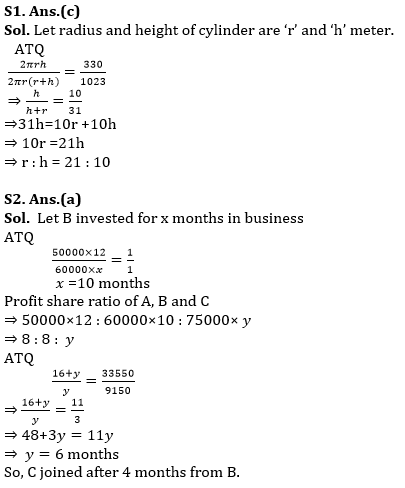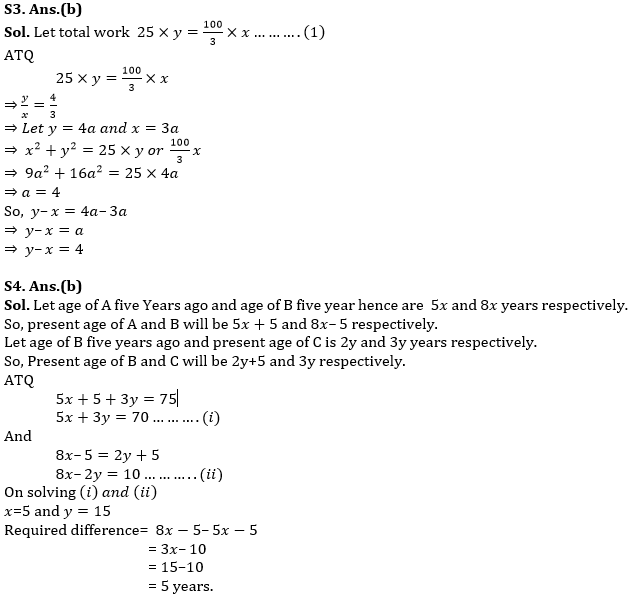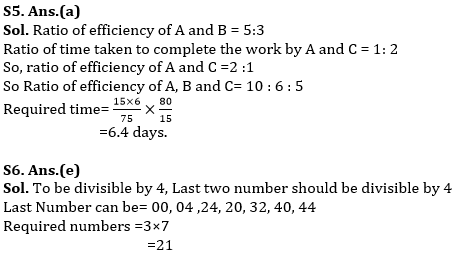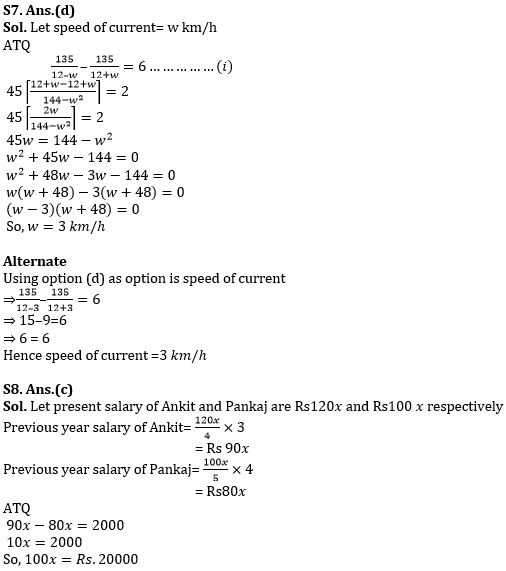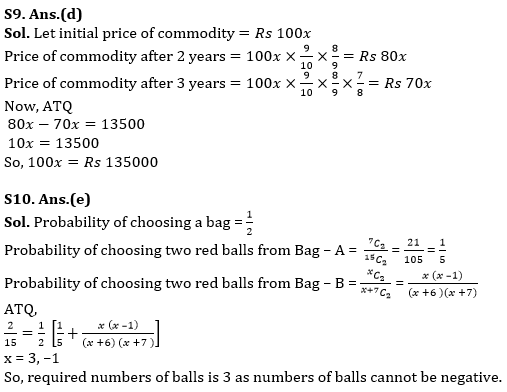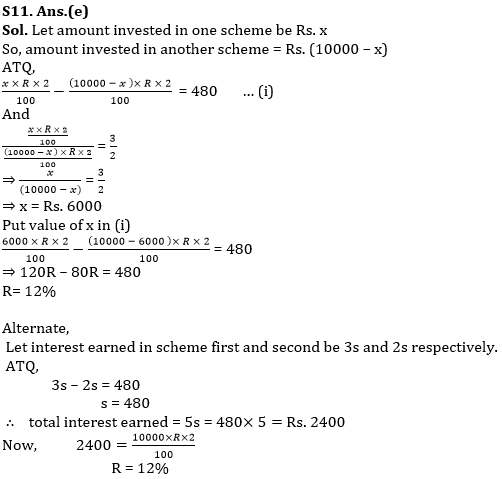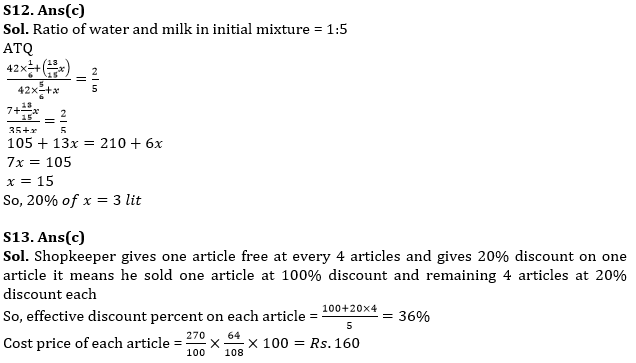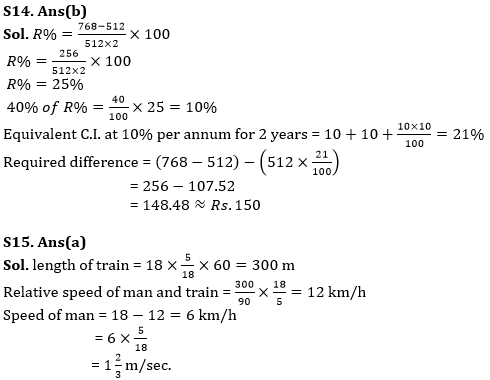Latest Banking jobs   »

# Quantitative Aptitude Quiz For RBI Assistant/ ESIC UDC Mains 2022- 01st April

Q1. If curved surface area and total surface area of a cylinder are 330m² and 1023m² respectively. Find ratio of radius to height of cylinder?
(a) 10 : 7
(b) 7 : 5
(c) 21 : 10
(d) 21 : 5
(e) Can’t be determined

Q2. A started a business with Rs 50000 and after some month B joined the business with Rs 60000. Again, after some month C joined the business with Rs 75000. At the end of the year C got Rs. 9150 out of total profit of Rs 33550 and profit share of A and B is equal. Find how many months after B joined the business did C join?
(a) 4 months
(b) 6 months
(c) 10 months
(d) 3 months
(e) none of these.

Q3. Two person of equal ability starts making a wall. First person works for x hours for x day and second person works for y hours for y days and they complete the wall. If first person works alone for it’s respective hours per day then it takes 33⅓ day to complete the wall and if second person works alone for it’s respective hours then it takes 25 days to complete the wall. Find (y–x)?
(a) 3
(b) 4
(c) 2
(d) 5
(e) 1

Q4. Sum of present age of A and C is 75 years. Ratio of age of A five years ago to age of B five years hence is 5:8, and ratio of age of B five years ago to present age of C is 2:3. Find difference between present age of A and B?
(a) 10 years
(b) 5 years
(c) 3 years
(d) 8 year
(e) 6 year

Q5. B is 40% less efficient than A who can complete the work in 50% less time than C. If 75% of total works can be completed in 15 day by B working alone then find in how many day A and C working together can complete the 80% of the work?
(a) 6.4 days
(b) 4 days
(c) None of these
(d) 4.6 days
(e) 6⅔ days

Q6. How many three-digit number which are divisible by 4 can be formed using 0,2,3,4, with repetition of numbers?
(a) 20
(b) 15
(c) 28
(d) 7
(e) 21

Q7. A boat takes 6 hours more to cover a distance of 135 km in upstream than in downstream, if speed of boat in still water is 12 km/h, then find speed of current?
(a) 2 km/h
(b) 7 km/h
(c) 5 km/h
(d) 3 km/h
(e) 5/2 km/h

Q8. Ratio of present salary of Ankit and Pankaj is 6:5 and ratio of their present salary to previous year salary is 4:3 and 5:4 respectively. If difference between previous year salary of Ankit and Pankaj is Rs.2000, then find present salary of Pankaj?
(a) Rs 24000
(b) Rs 18000
(c) Rs 20000
(d) Rs 16000
(e) Rs 21000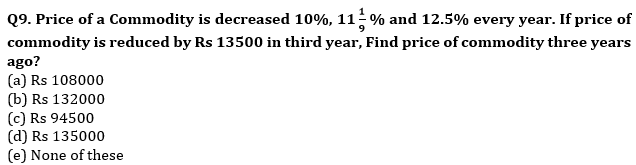Q10. Bag-A contains 6 blue balls, 7 red balls and 2 green balls and Bag-B contains 5 blue balls, x red balls and 2 green balls. A bag is chosen randomly and two balls are taken out from it randomly, then the probability of getting two red balls is 2/15. Find number of red balls in Bag-B.
(a) 2
(b) 5
(c) 1
(d) 4
(e) None of the above.

Q11. Dharam invested Rs.10000 in two schemes for two years and both schemes offer R% S.I. If difference between S.I. earned on both schemes is Rs.480 and ratio of interest earned from both schemes is 3 : 2. Then, find the value of R.
(a) 15 %
(b) 10 %
(c) 20 %
(d) 16 %
(e) None

Q12. In a mixture of 42 lit, water is 80% less than milk, if x lit of milk and 86⅔% ofx lit of water are mixed in the mixture respectively then ratio of water to milk become 2:5, find 20% of x?
(a) 5 lit
(b) 2 lit
(c) 3 lit
(d) 7 lit
(e) 1 lit

Q13. A shopkeeper gives 20% discount on each article. He also gives one article free at every 4 articles he sold and earns 8% profit, if M.R.P. of each article is Rs. 270 find cost price of one article when he bought five articles?
(a) Rs. 150
(b) Rs. 120
(c) Rs. 160
(d) Rs. 100
(e) None of these.

Q14. Hemant invested Rs. 512 at R% per annum at SI and received Rs. 768 as amount after two years, find how much he would loss (approximate), if he would have invested the same at 40% of R% for two years at CI? (compounded annually)
(a) Rs.200
(b) Rs.150
(c) Rs.175
(d) Rs.160
(e) Rs.125

Q15. A train is moving with 18 km/h and crosses a pole and a man moving in same direction in 1 min and 1.5 min respectively, find speed of the man?
(a) 1⅔ m/sec
(b) 6 m/sec
(c) 2 ⅔ m/sec
(d) 1⅓ m/sec
(e) none of these.

Solutions